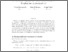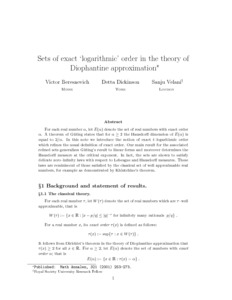# Sets of exact ‘logarithmic’ order in the theory of Diophantine approximation

Beresnevich, Victor and Dickinson, Detta and Velani, Sanju (2001) Sets of exact ‘logarithmic’ order in the theory of Diophantine approximation. Mathematische Annalen, 321 (2). pp. 253-273. ISSN 0025-5831Previewmore...Add this article to your Mendeley library

## Abstract

For each real number α, let E(α) denote the set of real numbers with exact order α. A theorem of Güting states that for α≥2 the Hausdorff dimension of E(α) is equal to 2/α. In this note we introduce the notion of exact t–logarithmic order which refines the usual definition of exact order. Our main result for the associated refined sets generalizes Güting's result to linear forms and moreover determines the Hausdorff measure at the critical exponent. In fact, the sets are shown to satisfy delicate zero-infinity laws with respect to Lebesgue and Hausdorff measures. These laws are reminiscent of those satisfied by the classical set of well approximable real numbers, for example as demonstrated by Khintchine's theorem.

Item Type: Article Cite as: Beresnevich, V., Dickinson, D. & Velani, S. Math Ann (2001) 321: 253. https://doi.org/10.1007/s002080100225 Real Number; Linear Form; Critical Exponent; Hausdorff Dimension Hausdorff Measure; Faculty of Science and Engineering > Mathematics and Statistics 10117 https://doi.org/10.1007/s002080100225 Dr. Detta Dickinson 18 Oct 2018 14:08 Mathematische Annalen Springer Verlag YesItem control page